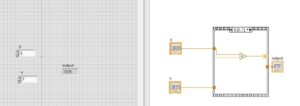Hello, readers welcome to the post. In this post, we will discuss How to use Sequence Structures in labview. In sequence there is one os more than one diagram called subdiagram or frames are applied in form sequence. In this every frame of sequence-structure in the remaining block diagram data values depend on the sequence of nodes performance

Most commonly used two main types of structure first one flate and second one stacked sequence structure. In this post we learn about the sequence structure to apply practically will make a project where structure is used. So let get started.

## How to use Sequence Structures in LabVIEW

• Here we will make two projects for flat and stack sequence-structure one by one with the details.

### Flate sequence Structure

• This sequence is used to show the all frame at a single time.
• It frames execution sequence is from left to right.
• It comes with features to resize by own
• Framas are arranged in sequence
• So now make a project to understand this flat structure practically.
• First of all, move to block diagram press right then structure and select the file structure according to these details.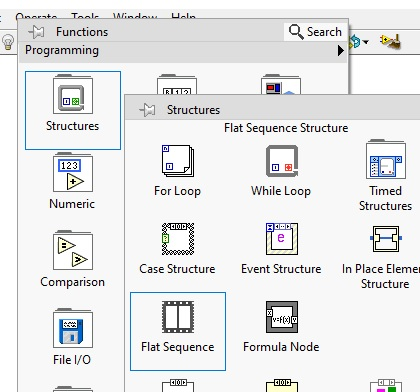• Practical look of flate sequence can seen here• there is more than frame can add in flate sequence in parallel combination for this press right on right side and choose option add frame after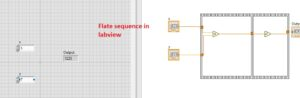• Now move to the front panel and choose two numeric control through passing right in front pannel and numeric option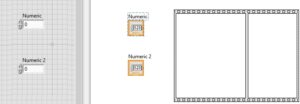• After that move to block diagram and press right then numeric and select the multiply and square tab and make configuration ash shown below.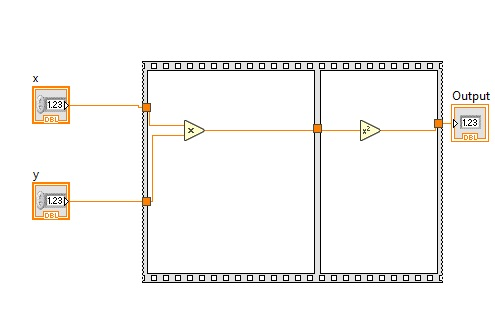• The final project that we have designed for flat sequence structure is shown here.
• In this projects, we have two inputs X and Y taking their multiply and after that output that multiplies of X and Y is then converted into a square.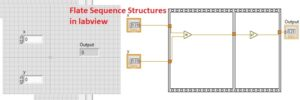• Here i have put the values and our resultant values of squares can see in the below diagram## Stacked Sequence structure

• In stacked sequence sturcture we will make a project as made for flate sequence and apply that practical to learn stacked sequence.
• To make a stacked sequence move to the block diagram press right then structure and select the flat sequence and make parallel frame like we above made.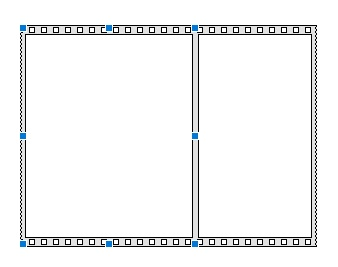• Now press right at the center of the above figure and you will see the option replaced with a stacked sequence option press on it.• Then you will see interfaces like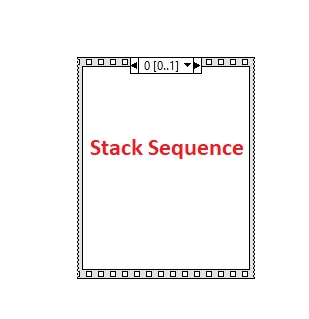• Now we will make the same projects as above made through using two numeric control in front panel and multiply tab and square tab in block diagram select all these components and place their positions. till now make circuit configuration as shown below after that there is som another process will apply to use the square option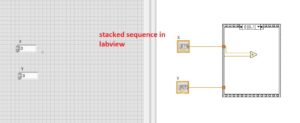• Now press right on frame and select the option add sequence local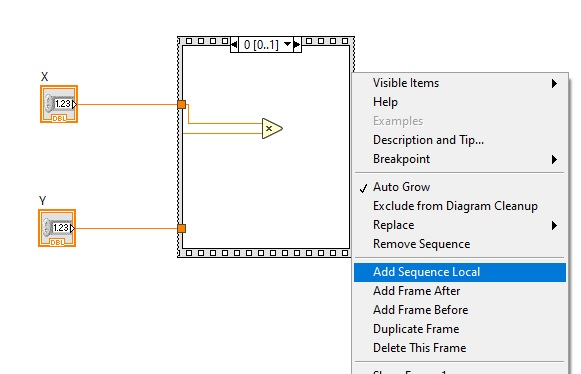• Now circuit will look like this• Now press at the arrow at top and change from 0 to 1 and circuit look like this• Now add square from numeric tab and make circuit connection as shown below.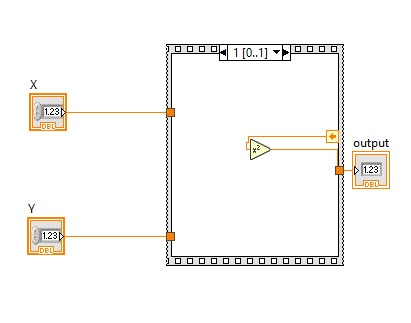Now run your program you will see the same result as we find in flate sequence.